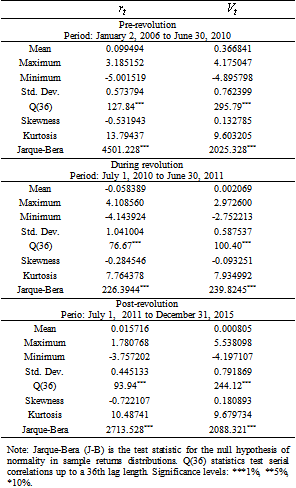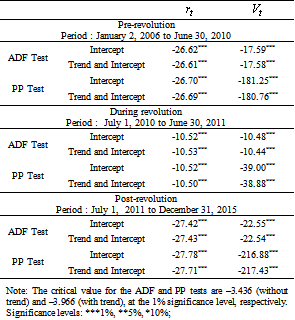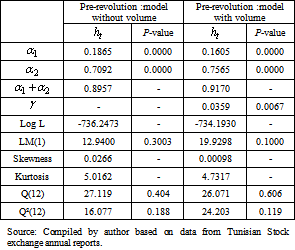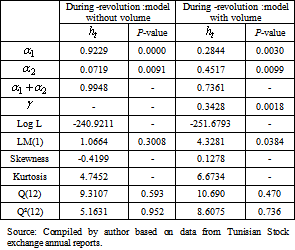## Tables index

#### Othman Mnari

International Journal of Global Energy Markets and Finance. 2019, 2(1), 1-5• Table 1. Descriptive statistics of return and volume for pre, during and post revolution• Table 2. Unit root tests for returns and trading volume• Table 3. Volatility persistence on the pre-revolution period: Maximum likelihood estimation of GARCH (1,1) model assuming the hypothesis of Generalized Error Distribution• Table 4. Volatility persistence during the revolution period: Maximum likelihood estimation of GARCH (1,1) model assuming the hypothesis of Generalized Error Distribution• Table 5. Volatility persistence on the post-revolution period: Maximum likelihood estimation of GARCH (1,1) model assuming the hypothesis of Generalized Error Distribution